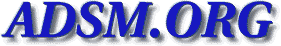nv-l [Top] [All Lists]

 ```Jim: Thank you for the greate details in your note. I have changed the "thewall=20480" from 16384. I will be testing this in the near future. Here a the of values associated with the no -a command. I do have 500 meg of memory so, let me know if you see something else that migth help. thewall = 20480 sb_max = 65536 somaxconn = 1024 clean_partial_conns = 0 net_malloc_police = 0 rto_low = 1 rto_high = 64 rto_limit = 7 rto_length = 13 arptab_bsiz = 7 arptab_nb = 25 tcp_ndebug = 100 ifsize = 8 arpqsize = 1 route_expire = 0 strmsgsz = 0 strctlsz = 1024 nstrpush = 8 strthresh = 85 psetimers = 20 psebufcalls = 20 strturncnt = 15 pseintrstack = 12288 lowthresh = 90 medthresh = 95 psecache = 1 subnetsarelocal = 1 maxttl = 255 ipfragttl = 60 ipsendredirects = 1 ipforwarding = 0 udp_ttl = 30 tcp_ttl = 60 arpt_killc = 20 tcp_sendspace = 16384 tcp_recvspace = 16384 udp_sendspace = 9216 udp_recvspace = 41600 rfc1122addrchk = 0 nonlocsrcroute = 0 tcp_keepintvl = 150 tcp_keepidle = 14400 bcastping = 0 udpcksum = 1 tcp_mssdflt = 512 icmpaddressmask = 0 tcp_keepinit = 150 ie5_old_multicast_mapping = 0 rfc1323 = 0 pmtu_default_age = 10 pmtu_rediscover_interval = 30 udp_pmtu_discover = 0 tcp_pmtu_discover = 0 ipqmaxlen = 100 directed_broadcast = 1 ipignoreredirects = 0 ipsrcroutesend = 1 ipsrcrouterecv = 0 ipsrcrouteforward = 1 tcp_timewait = 1 Gil Irizarry 280 King of Prussia Road ST. Davids, PA 19087 Phone: (610) 902-3426 INTERNET: IRIZARRG AT LABS.WYETH DOT COM ```